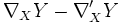# Riemannian submanifold

## Definition

Let$M$ be a differential manifold and$N$ a submanifold of$M$. Let$g$ be a Riemannian metric on$M$ turning$M$ into a Riemannian manifold. Then, since each tangent space to$N$ is a subspace ofa tangentspace to$M$, we can restrict$g$ to the tangent space to$N$ at each point. Further, the restriction will again be a smoothly varying positive definite symmetric bilinear form, and thus$N$ acquires the structure of a Riemannian manifold.$N$ equipped with this Riemannian manifold structure is termed a Riemannian submanifold.

## Levi-Civita connection on submanifold

The Levi-Civita connection on$N$ is not the same as that on$M$. However, it is almost the same, in the sense that if$\nabla$ and$\nabla'$ denote the Levi-Civita connections on$M$ and$N$ respectively, then for$X$ and$Y$ vector fields on$N$:$\nabla_XY - \nabla'_XY$

is, at each point, a vector normal to the submanifold$N$ in$M$. Thus$\nabla'$ is the component of$\nabla$ on the submanifold.

## Metric space structure to the submanifold

A Riemannian manifold naturally has the structure of a metric space: the distance between two points being the infimum of the lengths of paths between them. This metric space structure is particularly useful for a complete Riemannian manifold, which is thus a geodesic metric space. A submanifold of a Riemannian manifold can thus be given two metric space structures:

• One, the metric space structure obtained by restricting the metric from the bigger manifold
• Two, the metric space structure obtained from the Riemannian metric on the submanifold

Distances in the second metric space structure are in general greater, because there may be paths in the biger manifold shorter than the shortest path in the smaller manifold. The two metric space structures coincide only for a totally geodesic submanifold.